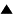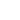The Principle of Asymmetric Transitions# The Principle of Asymmetric Transitions

 A transition from an unstable configuration to a stable one is possible, but the converse is not
This principle implies a fundamental asymmetry in evolution: one direction of change (from unstable to stable) is more likely than the opposite direction. The generalized, "continuous" version of the principle is the following:

The probability of transition from a less stable configuration A to a more stable one B is larger than the probability for the inverse transition: P (A -> B) > P (B -> A) (under the condition P (A -> B) =/ 0)

A similar principle was proposed by Ashby in his Principles of the Self-Organizing System (1962):"We start with the fact that systems in general go to equilibrium. Now most of a system's states are non-equilibrial [...] So in going from any state to one of the equilibria, the system is going from a larger number of states to a smaller. In this way, it is performing a selection, in the purely objective sense that it rejects some states, by leaving them, and retains some other state, by sticking to it. "

This reduction in the number of reachable states signifies that the variety, and hence the statistical entropy, of the system diminishes. It is because of this increase in neguentropy or organization that Ashby calls the process self-organization. But how does this fit in with the 2nd law of thermodynamics, which states that entropy in closed systems cannot decrease? The easy way out is to conclude that such a self-organizing system cannot be closed, and must lose entropy to its environment (von Foerster, 1960).

A deeper understanding can be reached by going back from the statistical definition of entropy to the thermodynamic one, in terms of energy or heat. Energy is defined as the capacity to do work, and working means making changes, that is to say exerting variation. Hence energy can ve viewed as potential variation. A stable configuration does not undergo variation. In order to destroy a stable equilibrium, you need to add energy, and the more stable the configuration, the more energy you will need. Therefore stability is traditionally equated with minimal energy.

The 1st law of thermodynamics states that energy is conserved. A naive interpretation of that law would conclude that the principle of asymmetric transitions cannot be valid, since it postulates a transition from an unstable (high energy) to a stable (low energy) configuration. If energy is absolutely conserved, then an unstable configuration can only be followed by another unstable configuration. This is the picture used in classical mechanics, where evolution is reversible, that is to say symmetric. Incidentally, this shows that the principle of asymmetric transitions is not tautological - though it may appear self-evident - , since a perfectly consistent theory (classical mechanics) can be built on its negation.

Thermodynamics has enlarged that picture by allowing energy dissipation. But what happens with the "dissipated" energy? A simple model is provided by a quantum system (e.g. an electron bound in an atom) with its set of - usually discrete - energy levels. A configuration at a higher level will spontaneously fall down to a lower level, emitting a photon which carries the surplus energy away. In order to bring back the electron to its higher level, energy must be added by having a photon of the right energy and direction hit the electron, a rather improbable event. Hence, the low level can be viewed as a stable configuration, with a small probability of transition.

The conjunction of energy conservation and asymmetric transitions implies that configurations will tend to dissipate energy (or heat) in order to move to a more stable state. For a closed system, this is equivalent to the thermodynamical interpretation of the 2nd law, but not to the statistical one, as the statistical entropy can decrease when transition probabilities are asymmetric. In an open system, on the other hand, where new energy is continuously added, the configuration will not be able to reach the minimum energy level. In that case we might assume that it will merely tend to maximally dissipate incoming energy, since transitions where energy is emitted are (much) more probable than transitions where energy is absorbed. That hypothesis seems equivalent to the Law of maximum entropy production (Swenson, 19), which describes dissipative structures and other far-from-equilibrium configurations. In such configurations the stability is dynamic, in the sense that what is maintained is not a static state but an invariant process.

Such an application of the principle of asymmetric transitions is opposite to the most common interpretation of the 2nd law, namely that disorder and with it homogeneity tend to increase. In the present view, configurations tend to become more and more stable, emitting energy in the process. This might be seen as a growing differentiation between the negative energy of stable bonds, and the positive energy of photons and movement. Recent cosmological theories hypothesize a similar spontaneous separation of negative and positive energies to account for the creation of the universe out of a zero-energy vacuum (Hawking, 1988).

Reference: Heylighen F. (1992): "Principles of Systems and Cybernetics: an evolutionary perspective", in: Cybernetics and Systems '92, R. Trappl (ed.), (World Science, Singapore), p. 3-10.

 HomeMetasystem Transition TheoryPrinciples of Systems and CyberneticsBlind Variation and Selective Retention Up Prev.Next Down Definition of Fitness in terms of transition probabilities